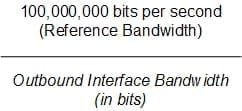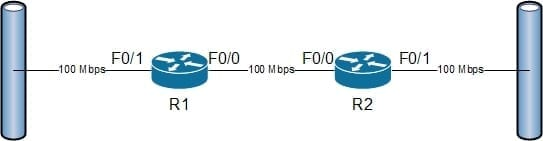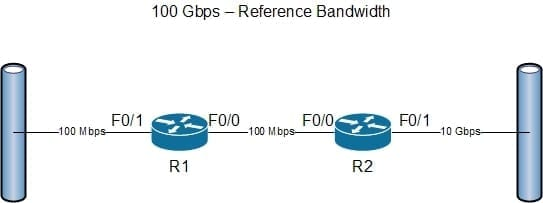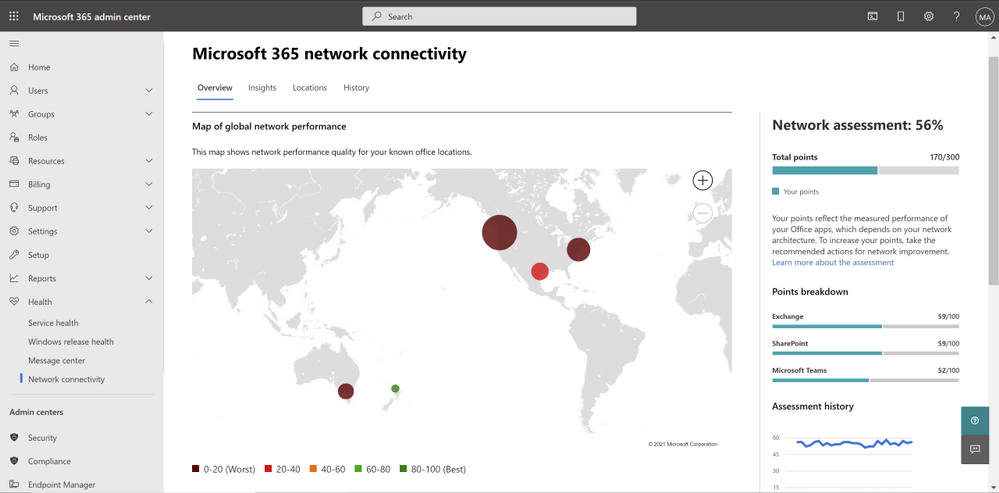close

Don't miss out on our next GET-IT conference about Virtual Desktop Infrastructure!

# OSPF Metrics Troubleshooting## Overview

When implementing any routing protocol, it is vital to have a handle on how the protocol operates and makes decisions. Without this knowledge, it is almost impossible to check and make sure that the protocol is configured properly and is operating as expected. This article takes a look at the Open Shortest Path First (OSPF) routing protocol, how its metric is calculated, and how this information can be used to ensure that traffic is taking the path that is expected.

## OSPF Metric Calculation

OSPF has one of the easiest metric calculations; by default, the bandwidth of the outbound interface is used to calculate each part of the route path. The default formula is shown in Figure 1:Figure 1 – OSPF Metric Formula

For example, a network contained two routers that were connected together, as shown in Figure 2:Figure 2 – OSPF Metric Sample Topology

Assuming that OSPF is configured, R1 would have an OSPF routing table entry for the network that is connected to R2’s F0/1 interface. For traffic from R1 to reach that network it would need to pass through both R1’s F0/0 interface and R2’s F0/1 interface. R2 would calculate the OSPF metric for its F0/1 interface (100,000,000 / 100,000,000 = 1) and R1 would calculate the OSPF metric for its F0/0 interface (100,000,000 / 100,000,000 = 1). Based on this information from R1’s perspective, the OSPF metric to the network off of R2’s F0/1 interface is 2.

It is very important to note that the bandwidth that OSPF is using in its metric calculations is based on the configured interface bandwidth using the bandwidth interface configuration mode command. The bandwidth that is configured with the bandwidth command does not have to match the physical bandwidth of the interface, and does not affect the physical bandwidth of the network. If the network administrator changed the bandwidth of R2’s F0/1 interface to 50 Mbps (bandwidth 50000) the metric for the OSPF route would change on R1, specifically, it would change to 3 (100,000,000 / 50,000,000) = 2 + 1 (R1’s F0/0 OSPF metric) = 3.

Another common issue that is found by network engineers in modern networks is that the reference bandwidth used in the OSPF metric calculation is rather small with the availability of 1, 10 and 100 gigabit interfaces. From the perspective of the OSPF metric, an interface with a bandwidth of 100 Mbps (1) has the same metric as one with a bandwidth of 100 Gbps (1) (The OSPF metric calculation only uses whole numbers). To remedy this, it is possible to change the reference bandwidth that the OSPF process is using for metric calculation. To change this, use the auto-cost reference-bandwidth reference-bandwidth command, where reference-bandwidth is set in Mbps (i.e. the default is 100). Make note that the reference bandwidth must be changed on ALL of the devices in the OSPF network.

To make this a little clearer, Figure 3 shows the same network using up-to-date interface bandwidths and a higher reference bandwidth:Figure 3 – OSPF Reference Bandwidth Example

Using these bandwidths, the OSPF metric to the network off of R2’s F0/1 interface would be calculated as follows:

R1’s F0/0 interface – 100,000,000,000 / 100,000,000 = 1,000

R2’s F0/1 interface – 100,000,000,000 / 10,000,000,000 = 10

R1’s routing table will have an entry for R2’s F0/1 network with a metric of 1010.

## Summary

Most of the time when routing protocols are implemented on small simple network topologies, they work without much additional configuration. When working on networks that are larger, the complexity of the routing protocol configuration can increase; this complexity and the size of the topology can make troubleshooting very complex as well. The OSPF metric is very simple to calculate and allows even novice engineers the ability to easily trace how traffic should pass through a network. Take the time to memorize how these metrics are calculated and future troubleshooting will become easier even on complex networks.

More in NetworkingUnderstanding Network Basics: What is TCP, Subnetting, and More

Sep 2, 2022 | Siji RoyStatic vs Dynamic IP Address - What's the Difference?

Mar 29, 2022 | Jason WynnWhat is DNS?

Mar 11, 2022 | Siji RoyWhat is 5G and How is it Better Than 4G LTE?

Feb 24, 2022 | Jason WynnWhat is a DHCP Server?

Dec 7, 2021 | Jason WynnHow to Access and Triage Network Connectivity in the Microsoft 365 Admin Center

May 19, 2021 | Michael Reinders

Most popular on petri

Article saved!

Access saved content from your profile page. View Saved